Previous: Contents   | Next: Tables

## Engenetics Diagrams

Figure 1: Defining ecology
from Chapter 2: Prolegomenon II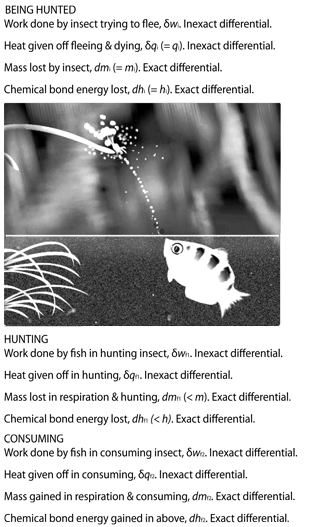Figure 2: Inertia and Force: A Marble in Motion
from Chapter 6: The importance of defining terms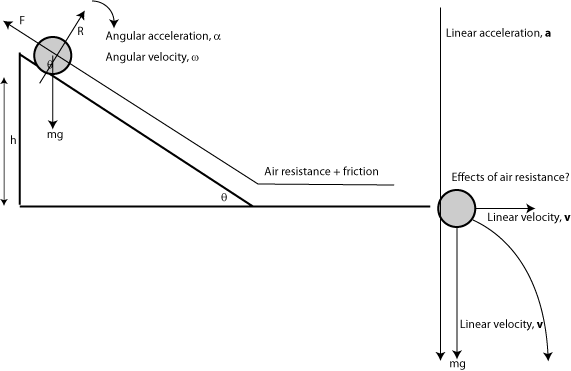Figure 3: Inertia and Force: Collision of an Air Mass
from Chapter 8: Biology and ecology lack core variables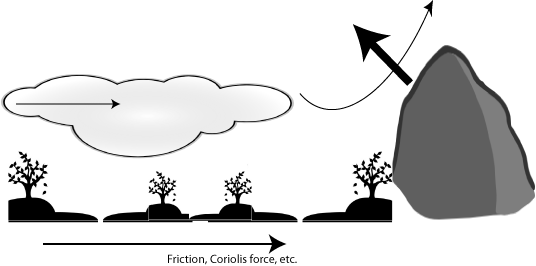Figure 4: Inertia and Force: Cold Fronts and Warm Fronts
from Chapter 8: Biology and ecology lack core variablesFigure 5: Velocity vector field for San Francisco Bay wind pattern
from Chapter 8: Biology and ecology lack core variables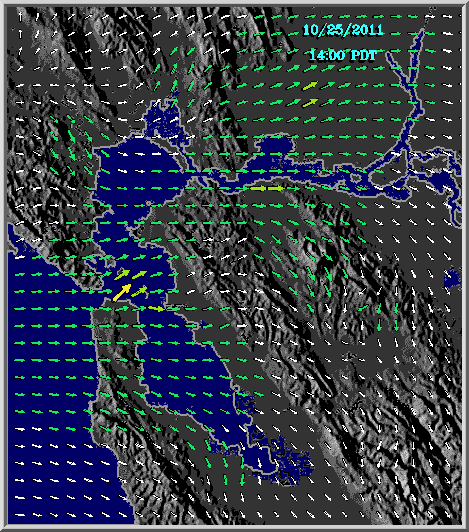Figure 6: Equivalence of Rectangle and Parallelogram
from Chapter 10: The planimeter: the instrument that measures natural selection and Darwinian evolution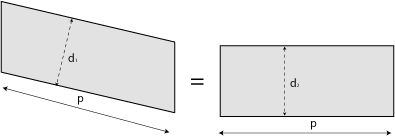Figure 7: Rectangular Addition and Subtraction
from Chapter 10: The planimeter: the instrument that measures natural selection and Darwinian evolution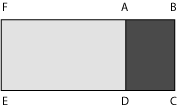Figure 8: Planimeter & Rectangle (1)
from Chapter 10: The planimeter: the instrument that measures natural selection and Darwinian evolutionFigure 9: Planimeter & Rectangle (2)
from Chapter 10: The planimeter: the instrument that measures natural selection and Darwinian evolution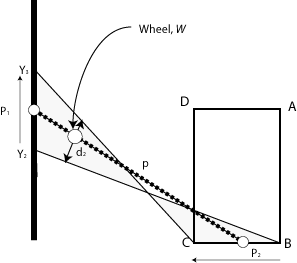Figure 10: Planimeter & Rectangle (3)
from Chapter 10: The planimeter: the instrument that measures natural selection and Darwinian evolutionFigure 11: Planimeter and Curved Area
from Chapter 10: The planimeter: the instrument that measures natural selection and Darwinian evolution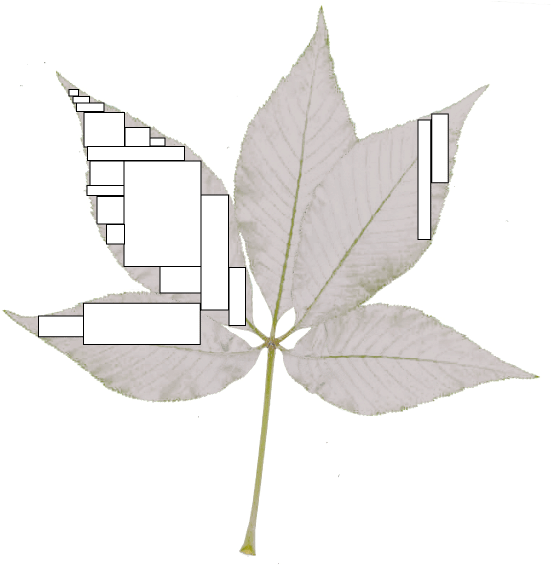Figure 12: Planimeter: Green's Theorem in the Plane
from Chapter 10: The planimeter: the instrument that measures natural selection and Darwinian evolution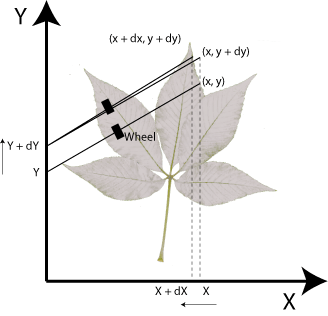Figure 13: Deep Structure: Some Examples of Isomorphism
from Chapter 13: A deep structure for biologyFigure 14: The Joule Experiment
from Chapter 14: Biology, ecology, molecules, and a volume of ignoranceFigure 15: The Helmholtz energy
from Chapter 16: Darwin's statement of the problem-the attacks upon his answerFigure 16: Competition & Natural Selection
from Chapter 19: A better way to the answerFigure 17: Towards a Biological Flux
from Chapter 21: The first maxim of ecology: the maxim of dissipation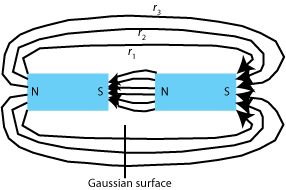Figure 18: Divergences in Biological Matter
from Chapter 21: The first maxim of ecology: the maxim of dissipation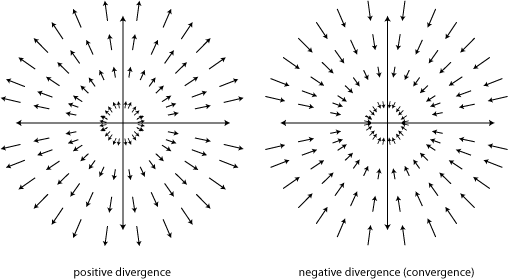Figure 19: An Electric Field
from Chapter 21: The first maxim of ecology: the maxim of dissipation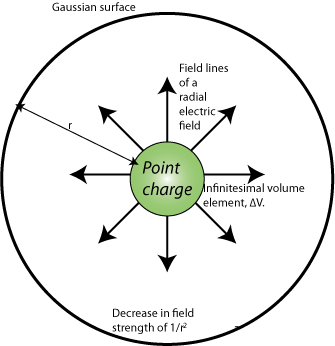Figure 20: The Capturing and Escaping Tendencies
from Chapter 22: The second maxim of ecology: the maxim of number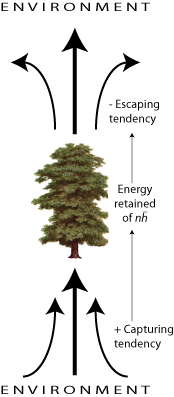Figure 21: Natural Selection & Competition
from Chapter 22: The second maxim of ecology: the maxim of number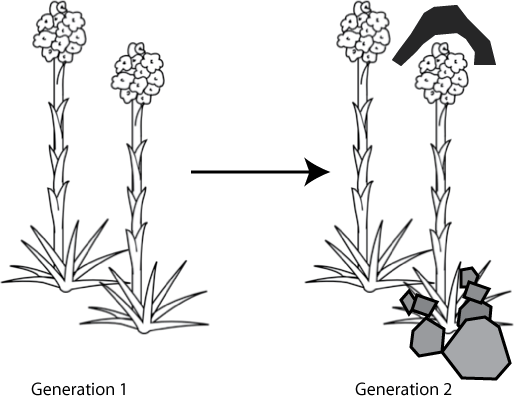Figure 22: Galileo's Thought Experiment
from Chapter 23: Darwin and Aristotle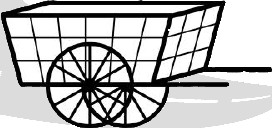Figure 23: A Three-Coordinate System
from Chapter 24: The origins for species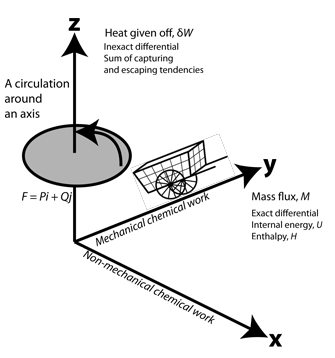Figure 24: Towards Biological Gradients
from Chapter 24: The origins for species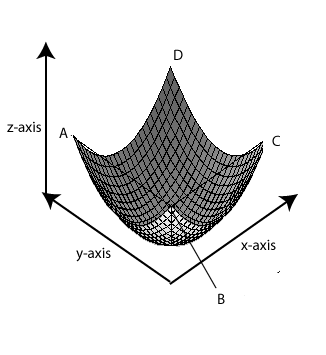Figure 25: A Gaussian Surface
from Chapter 25: The three constraints upon biological populations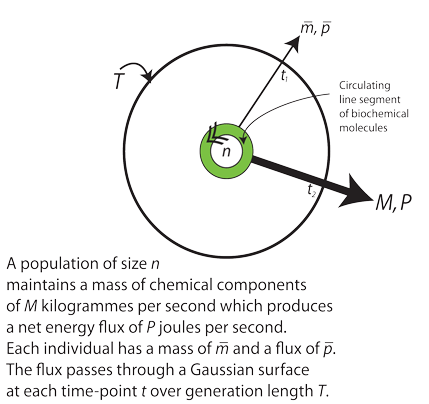Figure 26: Constant Volume: Non-Mechanical Chemical Energy
from Chapter 27: Discovering the energy of natural selection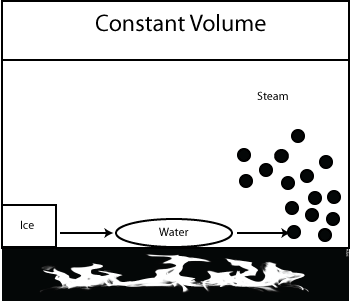Figure 27: Constant Pressure: Mechanical Chemical Energy
from Chapter 27: Discovering the energy of natural selection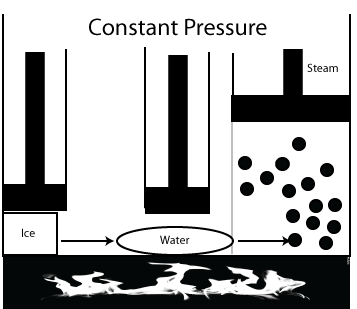Figure 28: Biological Potentiation
from Chapter 28: Discovering the forces behind natural selection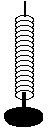Figure 29: The Simplest Possible Biological Population
from Chapter 30: Natural selection is a vector and a force of nature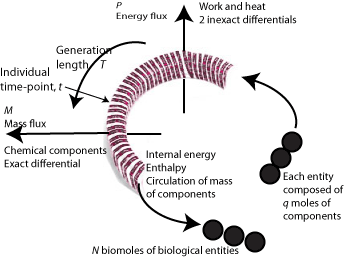Figure 30: The Intrinsic and the Extrinsic
from Chapter 31: The range of natural selectionFigure 31: The Volume of a Biological Population
from Chapter 31: The range of natural selection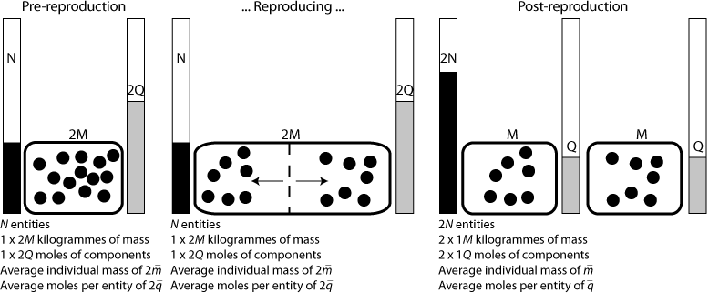Figure 32: Biological Mass and Biological Inertia
from Chapter 34: Natural selection and evolution: a mass misconceptionFigure 33: The Biot-Savart Law
from Chapter 35: Natural selection is put on the line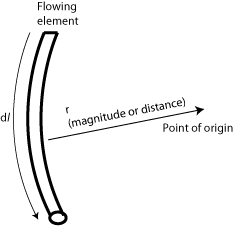Figure 34: Biological Induction: The Heritability of Darwinian Evolution
from Chapter 37: Darwinian evolution as biological inductionFigure 35: The Temporal Continuity of the Generations
from Chapter 37: Darwinian evolution as biological inductionFigure 36: Divergence and flux density
from Chapter 37: Darwinian evolution as biological induction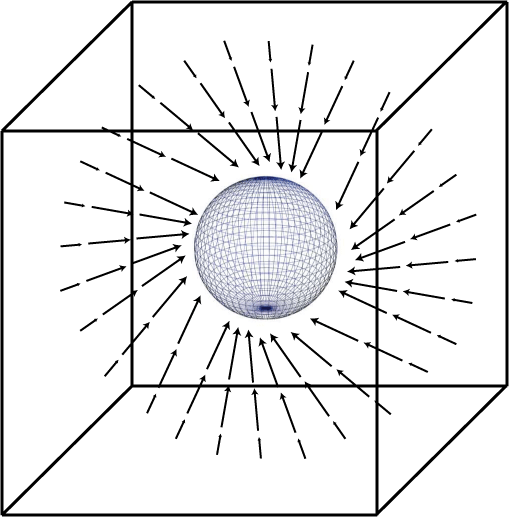Figure 37: Circulation and curl
from Chapter 37: Darwinian evolution as biological inductionFigure 38: Two-Dimensional Curl
from Chapter 37: Darwinian evolution as biological inductionFigure 39: New Perspectives
from Chapter 40: Natural selection: a power measured in wattsFigure 40: Open to Natural Selection
from Chapter 40: Natural selection: a power measured in watts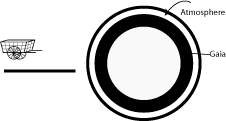Figure 41: Energy and Temperature
from Chapter 40: Natural selection: a power measured in wattsFigure 42: The Engeny of Species
from Chapter 40: Natural selection: a power measured in watts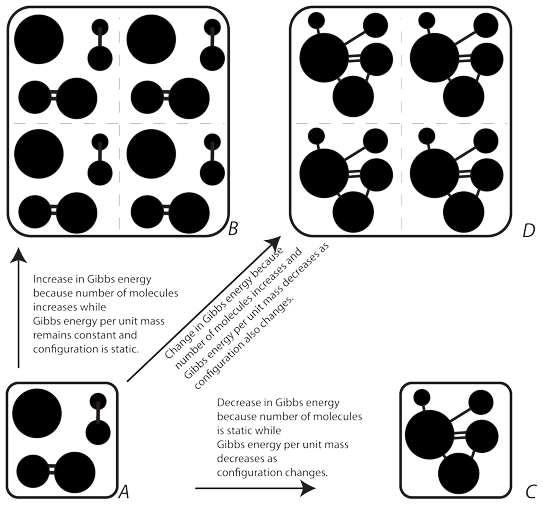Figure 43: The Generation Length
from Chapter 44: A time scale for natural selectionFigure 44: The Variation and Diversity of Natural Selection
from Chapter 45: Step one in defining a species: measuring slight variations: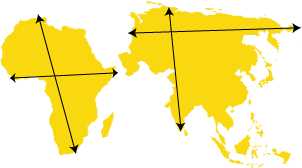Figure 45: Slight Variations
from Chapter 45: Step one in defining a species: measuring slight variations:Figure 46: Polar coordinates
from Chapter 45: Step one in defining a species: measuring slight variations: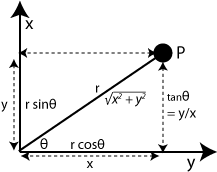Figure 47: Cylindrical coordinates
from Chapter 45: Step one in defining a species: measuring slight variations:Figure 48: Spherical coordinates
from Chapter 45: Step one in defining a species: measuring slight variations: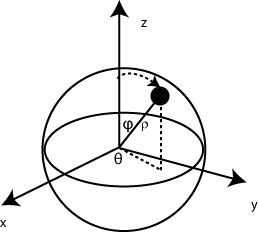Figure 49: Relative and Absolute Natural Selection
from Chapter 45: Step one in defining a species: measuring slight variations: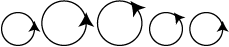Figure 50: The Variability of Natural Selection
from Chapter 45: Step one in defining a species: measuring slight variations: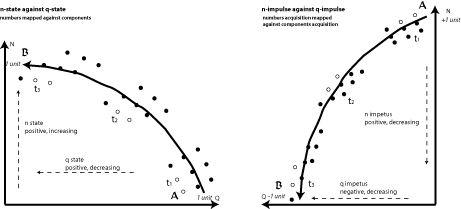Figure 51: The Force of Natural Selection
from Chapter 45: Step one in defining a species: measuring slight variations: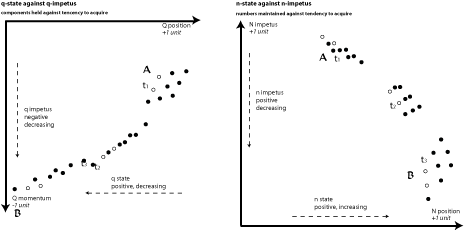Figure 52: Orientation for force
from Chapter 45: Step one in defining a species: measuring slight variations: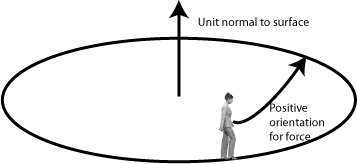Figure 53: The Mutability of Natural Selection
from Chapter 45: Step one in defining a species: measuring slight variations: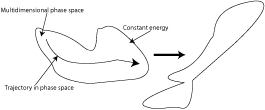Figure 54: The Equality of Natural Selection
from Chapter 46: Steps two and three in defining a species: the field of natural selection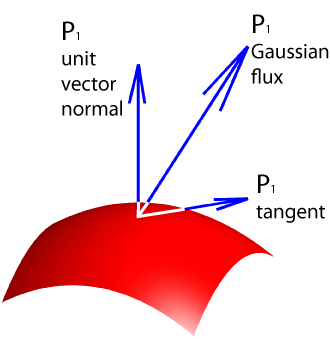Figure 55: Difference in Natural Selection
from Chapter 46: Steps two and three in defining a species: the field of natural selection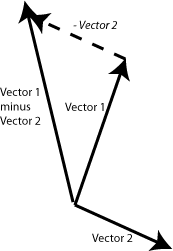Figure 56: Natural Selection about a boundary
from Chapter 48: The definition of a species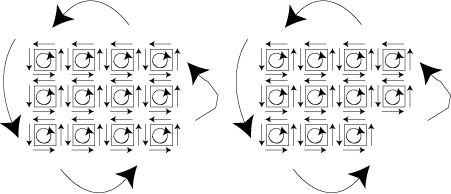Figure 57: The Mass and Components Flux
from Chapter 48: The definition of a speciesFigure 58: The Configuration of a Generation
from Chapter 48: The definition of a species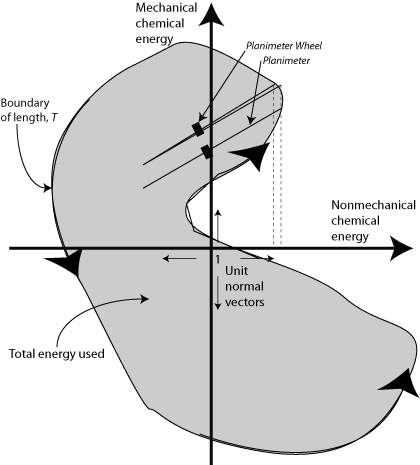Figure 59: The specification of a biological population
from Chapter 48: The definition of a species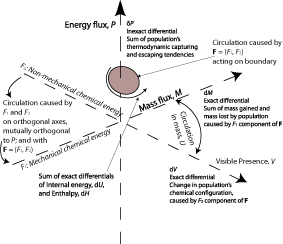Figure 60: The Circulation of the Generations
from Chapter 48: The definition of a species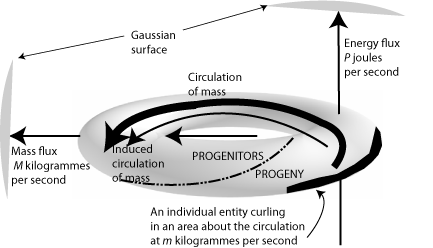Figure 61: The Joule experiment and the Rocket
from Chapter 50: The demonstration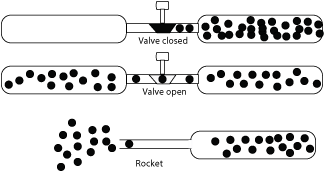Figure 62: The Generation Length
from Chapter 50: The demonstrationFigure 63: Energy and Work
from Chapter 50: The demonstration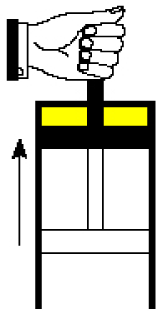Figure 64: The Perpetual Motion Machine
from Chapter 50: The demonstration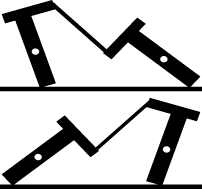Figure 65: Biology and the Biot-Savart law
from Chapter 51: Conclusion: A general theory of biologyFigure 66: Worldlines and light cones
from Chapter 51: Conclusion: A general theory of biology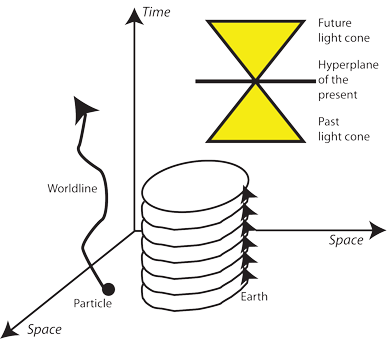Figure 67: Worldline, worldsheet, worldvolume
from Chapter 51: Conclusion: A general theory of biology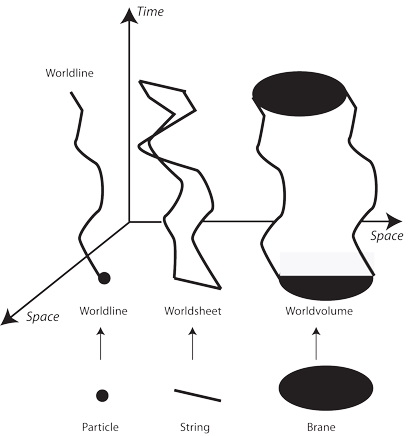Figure 68: A biotrail and a biopath within a biological field
from Chapter 51: Conclusion: A general theory of biology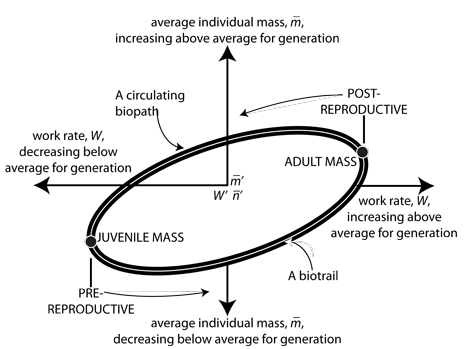Figure 69: Quantum biology: the particle and the wave
from Chapter 51: Conclusion: A general theory of biology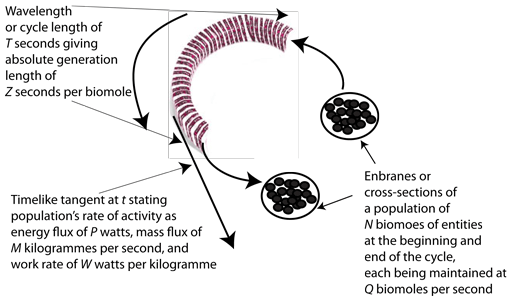Figure 70: The Franklin cycle
from Chapter 51: Conclusion: A general theory of biologyFigure 71: Reproductive isolation
from Chapter 51: Conclusion: A general theory of biology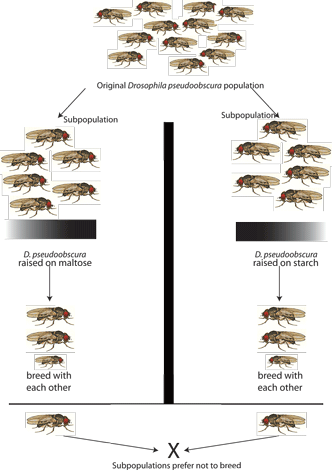Figure 72: Towards the general theory for biology
from Chapter 51: Conclusion: A general theory of biology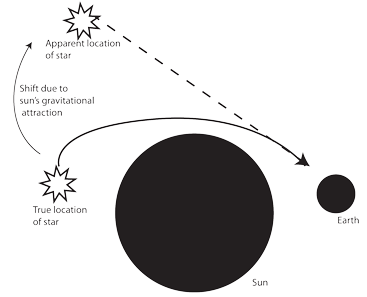Figure 73: Energy density and the Franklin cycle
from Chapter 51: Conclusion: A general theory of biologyFigure 74: Constructing a metric for energy density
from Chapter 51: Conclusion: A general theory of biologyFigure 75: A Riemannian manifold for biology
from Chapter 51: Conclusion: A general theory of biologyFigure 76: Shear stresses
from Chapter 51: Conclusion: A general theory of biology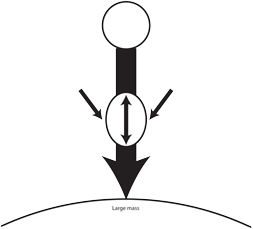Figure 77: A normal environment
from Chapter 51: Conclusion: A general theory of biologyFigure 78: Biology, ecology, and parallel transport
from Chapter 51: Conclusion: A general theory of biology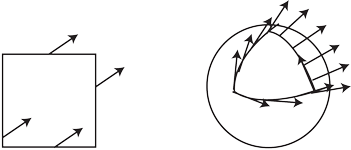Figure 79: The cone of reproductive accessibility
from Chapter 51: Conclusion: A general theory of biology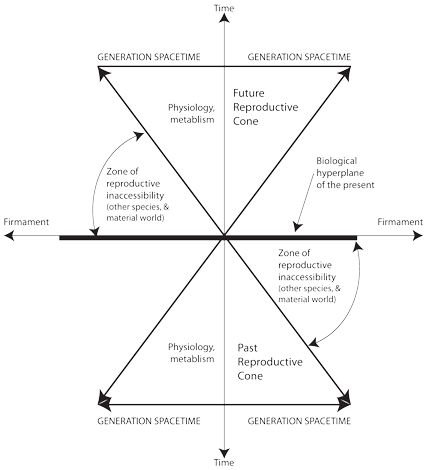Figure 80: Timelike and spacelike
from Chapter 51: Conclusion: A general theory of biology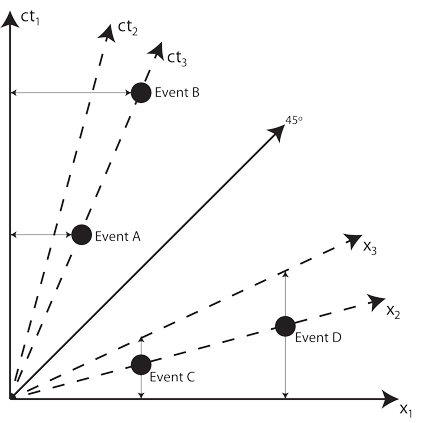Figure 81: Round the generation: a polar planimeter
from Chapter 51: Conclusion: A general theory of biology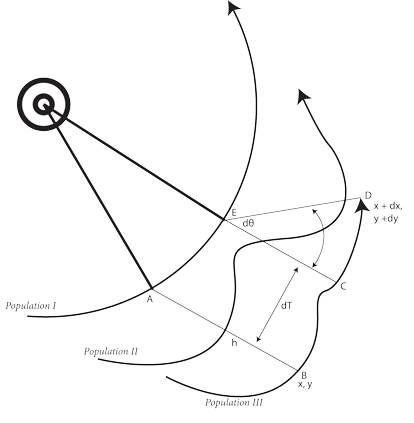Figure 82: The origin of biological field lines
from Chapter 51: Conclusion: A general theory of biology